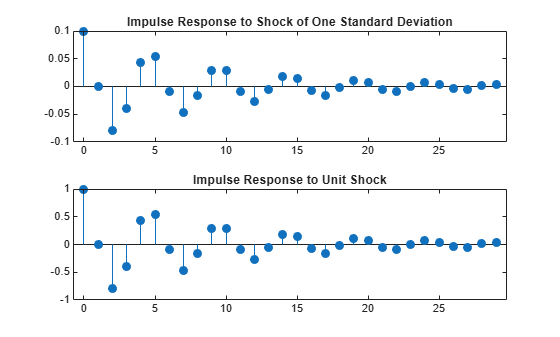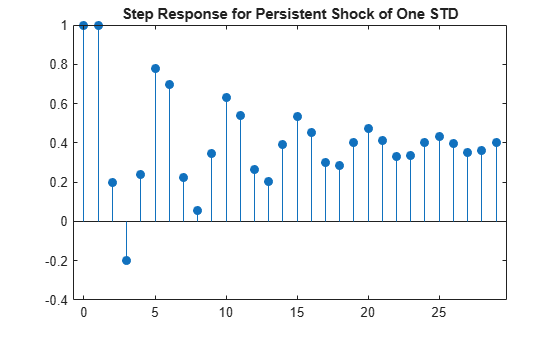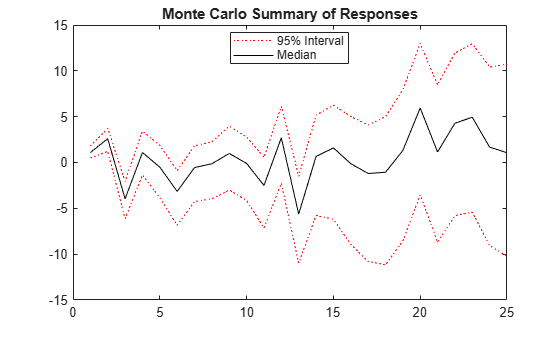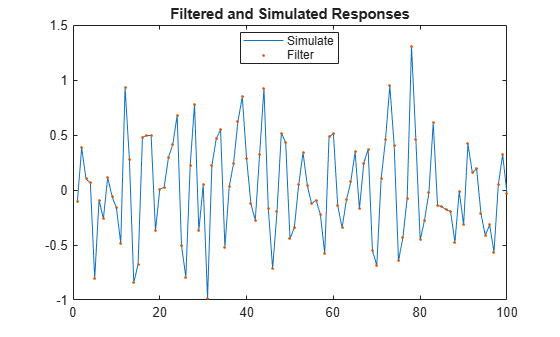# filter

Filter disturbances through regression model with ARIMA errors

## Syntax

Y = filter(Mdl,Z)
Y = filter(Mdl,Z,Name=Value)
[Y,E,U] = filter(___)

## Description

example

Y = filter(Mdl,Z) returns one or more observed response series Y resulting from filtering one or more error series Z, each drives the innovation process, through the univariate regression model with ARIMA errors Mdl.

example

Y = filter(Mdl,Z,Name=Value) uses additional options specified by one or more name-value arguments. For example, filter(Mdl,Z,X=Pred,Z0=PresampleErrors) specifies the predictor data Pred for the model regression component and the observed errors in the presample period PresampleErrors to initialize the model.

example

[Y,E,U] = filter(___) also returns one or more innovations and unconditional disturbance series, which are E and U, respectively, using any input argument combination in the previous syntaxes.

## Examples

collapse all

Compute the impulse response function of an innovation shock to the regression model with ARMA(2,1) errors.

The impulse response assesses the dynamic behavior of a system to a one-time shock. Typically, the magnitude of the shock is 1. Alternatively, it might be more meaningful to examine an impulse response of an innovation shock with a magnitude of one standard deviation.

In regression models with ARIMA errors,

• The impulse response function is invariant to the behavior of the predictors and the intercept.

• The impulse response of the model is defined as the impulse response of the unconditional disturbances as governed by the ARIMA error component.

Specify the following regression model with ARMA(2,1) errors:

$\begin{array}{c}{y}_{t}={u}_{t}\\ {u}_{t}=0.5{u}_{t-1}-0.8{u}_{t-2}+{\epsilon }_{t}-0.5{\epsilon }_{t-1},\end{array}$

where ${\epsilon }_{t}$ is Gaussian with variance 0.1.

Mdl = regARIMA(Intercept=0,AR={0.5 -0.8},MA=-0.5, ... Variance=0.1);

When you construct an impulse response function for a regression model with ARIMA errors, you must set Intercept to 0.

Simulate the first 30 responses of the impulse response function by generating a error series with a one-time impulse with magnitude equal to one standard deviation, and then filter it. Also, use impulse to compute the impulse response function.

z = [sqrt(Mdl.Variance); zeros(29,1)]; % Shock of 1 std yFltr = filter(Mdl,z); yImpls = impulse(Mdl,30);

When you construct an impulse response function for a regression model with ARIMA errors containing a regression component, do not specify the predictor matrix, X, in filter.

Plot the impulse response functions.

figure subplot(2,1,1) stem((0:numel(yFltr)-1)',yFltr,"filled") title("Impulse Response to Shock of One Standard Deviation") subplot(2,1,2) stem((0:numel(yImpls)-1)',yImpls,"filled") title("Impulse Response to Unit Shock")The impulse response function given a shock of one standard deviation is a scaled version of the impulse response returned by impulse.

Simulate the step response function of a regression model with ARMA(2,1) errors.

The step response assesses the dynamic behavior of a system to a persistent shock. Typically, the magnitude of the shock is 1. Alternatively, it might be more meaningful to examine a step response of a persistent innovation shock with a magnitude of one standard deviation. This example plots the step response of a persistent innovations shock in a model without an intercept and predictor matrix for regression. However, note that filter is flexible in that it accepts a persistent innovations or predictor shock that you construct using any magnitude, then filters it through the model.

Specify the following regression model with ARMA(2,1) errors:

$\begin{array}{c}{y}_{t}={u}_{t}\\ {u}_{t}=0.5{u}_{t-1}-0.8{u}_{t-2}+{\epsilon }_{t}-0.5{\epsilon }_{t-1},\end{array}$

where ${\epsilon }_{t}$ is Gaussian with variance 0.1.

Mdl = regARIMA(Intercept=0,AR={0.5 -0.8},MA=-0.5, ... Variance=0.1);

Simulate the first 30 responses to a sequence of unit errors by generating an error series of one standard deviation, and then filtering it.

z = sqrt(Mdl.Variance)*ones(30,1); % Persistent shock of one std y = filter(Mdl,z); y = y/y(1); % Normalize relative to y(1)

Plot the step response function.

figure stem((0:numel(y)-1)',y,"filled") title("Step Response for Persistent Shock of One STD")The step response settles around 0.4.

Simulate 100 independent paths of responses by filtering 100 independent paths of errors ${\mathit{z}}_{\mathit{t}}$, where innovations ${\epsilon }_{\mathit{t}}=\sigma \text{\hspace{0.17em}}{\mathit{z}}_{\mathit{t}}$, through the following regression model with SARIMA${\left(2,1,1\right)}_{12}$ errors.

$\begin{array}{c}{y}_{t}=X\left[\begin{array}{c}1.5\\ -2\end{array}\right]+{u}_{t}\\ \left(1-0.2L-0.1{L}^{2}\right)\left(1-L\right)\left(1-0.01{L}^{12}\right)\left(1-{L}^{12}\right){u}_{t}=\left(1+0.5L\right)\left(1+0.02{L}^{12}\right){\epsilon }_{t},\end{array}$

where ${\epsilon }_{t}$ follows a $\mathit{t}$-distribution with 15 degrees of freedom.

Distribution = struct("Name","t","DoF",15); Mdl = regARIMA(AR={0.2 0.1},SAR=0.01,SARLags=12, ... MA=0.5,SMA=0.02,SMALags=12,D=1,Seasonality=12, ... Beta=[1.5; -2],Intercept=0,Variance=0.1, ... Distribution=Distribution)
Mdl = regARIMA with properties: Description: "Regression with ARIMA(2,1,1) Error Model Seasonally Integrated with Seasonal AR(12) and MA(12) (t Distribution)" Distribution: Name = "t", DoF = 15 Intercept: 0 Beta: [1.5 -2] P: 27 D: 1 Q: 13 AR: {0.2 0.1} at lags [1 2] SAR: {0.01} at lag  MA: {0.5} at lag  SMA: {0.02} at lag  Seasonality: 12 Variance: 0.1 

Simulate a length 25 path of data from the standard bivariate normal distribution for the predictor variables in the regression component.

rng(1) % For reproducibility numObs = 25; Pred = randn(numObs,2);

Simulate 100 independent paths of errors of length 25 from the standard normal distribution.

numPaths = 100; Z = randn(numObs,numPaths);

Simulate 100 independent response paths from model by filtering the paths of errors through the model. Supply the predictor data for the regression component.

Y = filter(Mdl,Z,X=Pred); figure plot(Y) title('{\bf Simulated Response Paths}')Plot the 2.5th, 50th (median), and 97.5th percentiles of the simulated response paths.

lower = prctile(Y,2.5,2); middle = median(Y,2); upper = prctile(Y,97.5,2); figure plot(1:25,lower,"r:",1:25,middle,"k", ... 1:25,upper,"r:") title("Monte Carlo Summary of Responses") legend("95% Interval","Median",Location="best")Simulate responses using filter and simulate. Then compare the simulated responses.

Both filter and simulate filter a series of errors to produce output responses y, innovations e, and unconditional disturbances u. The difference is that simulate generates errors from Mdl.Distribution, whereas filter accepts a random array of errors that you generate from any distribution.

Specify the following regression model with ARMA(2,1) errors:

$\begin{array}{l}\begin{array}{c}{y}_{t}={X}_{t}\left[\begin{array}{c}0.1\\ -0.2\end{array}\right]+{u}_{t}\\ {u}_{t}=0.5{u}_{t-1}-0.8{u}_{t-2}+{\epsilon }_{t}-0.5{\epsilon }_{t-1},\end{array}\end{array}$

where ${\epsilon }_{t}$ is Gaussian with variance 0.1.

Mdl = regARIMA(Intercept=0,AR={0.5 -0.8},MA=-0.5, ... Beta=[0.1 -0.2],Variance=0.1);

Mdl is a fully specified regARIMA object.

Simulate a one path of bivariate standard normal data for the predictor variables. Then, simulate a path of responses and innovations from the regression model with ARMA(2,1) errors. Supply the simulated predictor data to simulate for the regression component.

rng(1) % For reproducibility Pred = randn(100,2); % Simulate predictor data [ySim,eSim] = simulate(Mdl,100,X=Pred);

ySim and eSIM are 100-by-1 vectors of simulated responses and innovations, respectively, from the model Mdl.

Produce model errors by standardizing the simulated innovations. Filter the simulated errors through the model. Supply the predictor data to filter.

z1 = eSim./sqrt(Mdl.Variance); yFlt1 = filter(Mdl,z1,X=Pred);

yFlt1 is a 100-by-1 vector of reponses resulting from filtering the simulated errors z1 through the model Mdl.

Confirm that the simulated responses from simulate and filter are identical by plotting the two series.

figure h1 = plot(ySim); hold on h2 = plot(yFlt1,"."); title("Filtered and Simulated Responses") legend([h1 h2],["Simulate" "Filter"],Location="best") hold offAlternatively, simulate responses by randomly generating your own errors and passing them into filter.

rng(1) Pred = randn(100,2); z2 = randn(100,1); yFlt2 = filter(Mdl,z2,X=Pred); figure h1 = plot(ySim); hold on h2 = plot(yFlt2,"."); title("Filtered and Simulated Responses") legend([h1 h2],["Simulate" "Filter"],Location="best") hold offThis plot is the same as the previous plot, confirming that both simulation methods are equivalent.

filter multiplies the error, Z, by sqrt(Mdl.Variance) before filtering Z through the model. Therefore, if you want to specify a different distribution, set Mdl.Variance to 1, and then generate your own errors using, for example, random("unif",a,b) for the Uniform(a, b) distribution.

## Input Arguments

collapse all

Fully specified regression model with ARIMA errors, specified as a regARIMA model object created by regARIMA or estimate.

The properties of Mdl cannot contain NaN values.

Error series zt that drives the innovations process εt, specified as a length numObs numeric column vector or a numObs-by-numPaths numeric matrix. numObs is the length of the time series (sample size). numPaths is the number of separate, independent disturbance paths. The innovations process εt = σzt, where σ = sqrt(Mdl.Variance), the standard deviation of the innovations.

Each row corresponds to a period. The last row contains the latest set of errors.

Each column corresponds to a separate, independent path of errors. filter assumes that errors across any row occur simultaneously.

Z is the continuation of the presample errors Z0.

Data Types: double

### Name-Value Arguments

Specify optional pairs of arguments as Name1=Value1,...,NameN=ValueN, where Name is the argument name and Value is the corresponding value. Name-value arguments must appear after other arguments, but the order of the pairs does not matter.

Before R2021a, use commas to separate each name and value, and enclose Name in quotes.

Example: filter(Mdl,Z,X=Pred,Z0=PresampleErrors) specifies the predictor data Pred for the model regression component and the observed errors in the presample period PresampleErrors to initialize the model.

Predictor data for the model regression component, specified as a numObs-by-numPreds numeric matrix.

numPreds is the number of predictor variables (numel(Mdl.Beta)).

Each row of X corresponds to the corresponding period in Z (period for which filter filters errors; the period after the presample). The following conditions apply:

• The last row contains the latest predictor data.

• If X has more then numObs rows, filter uses only the latest numObs rows.

• filter does not apply the regression component during the presample period.

Columns of X are separate predictor variables.

filter applies X to each filtered path; that is, X represents one path of observed predictors.

By default, filter excludes the regression component, regardless of its presence in Mdl.

Data Types: double

Presample error data providing initial values for the input error series Z, specified as a numeric column vector or a numeric matrix.

Each row of Z0 corresponds to a period in the presample. The following conditions apply:

• The last row contains the latest presample errors.

• To initialize the compound moving average (MA) component of the ARIMA error model, Z0 must have at least Mdl.Q rows.

• If Z0 has more rows than is required to initialize the model, filter uses only the latest required rows.

Columns of Z0 are separate, independent presample paths. The following conditions apply:

• If Z0 is a column vector, filter applies it to each path.

• If Z0 is a matrix, filter applies Z0(:,j) to initialize path j. Z0 must have at least numPaths columns; filter uses only the first numPaths columns of U0.

By default, filter sets the necessary presample errors to 0.

Data Types: double

Presample unconditional disturbances that provide initial values for the ARIMA error model, specified as a column vector or matrix.

Each row of U0 corresponds to a period in the presample. The following conditions apply:

• The last row contains the latest presample unconditional disturbances.

• To initialize the compound autoregressive (AR) component of the ARIMA error model, U0 must have at least Mdl.P rows.

• If U0 has more rows than is required to initialize the model, filter uses only the latest required rows.

Columns of U0 are separate, independent presample paths. The following conditions apply:

• If U0 is a column vector, filter applies it to each path.

• If U0 is a matrix, filter applies U0(:,j) to initialize path j. U0 must have at least numPaths columns; filter uses only the first numPaths columns of U0.

By default, filter sets the necessary presample unconditional disturbances to 0.

Data Types: double

Note

• NaNs in input data indicate missing values. filter uses listwise deletion to delete all sampled times (rows) in the input data containing at least one missing value. Specifically, filter performs these steps:

1. Synchronize, or merge, the presample data sets Z0 and U0 to create the set Presample, in other words, Presample = [Z0 U0].

2. Synchronize the filter data Z and X to create the set Data, in other words, Data = [Z X].

3. Remove all rows from Presample and Data containing at least one NaN.

Listwise deletion applied to the filter data can reduce the sample size and create irregular time series.

filter merges data sets that have a different number of rows according to the latest observation, and then pads the shorter series with enough default values to form proper matrices.

• filter assumes that you synchronize the data sets so that the latest observations occur simultaneously. The software also assumes that you synchronize the presample series similarly.

• All predictor variables (columns) in X are associated with each error series in Z to produce numPaths response series Y.

## Output Arguments

collapse all

Simulated response paths yt, returned as a length numObs column vector or a numObs-by-numPaths numeric matrix.

For each t = 1, …, numObs, the simulated responses at time t Y(t,:) correspond to the filtered errors at time t Z(t,:) and response path j Y(:,j) corresponds to the filtered disturbance path j Z(:,j) when Z is a matrix.

Y represents the continuation of presample inputs.

Simulated, mean-zero innovations paths εt of the model unconditional disturbances ut, returned as a length numObs column vector or a numObs-by-numPaths numeric matrix.

The dimensions of Y and E correspond.

Columns of E are scaled disturbance paths (innovations) such that, for a particular path, εt = σzt.

Simulated unconditional disturbance paths ut, returned as a length numObs column vector or a numObs-by-numPaths numeric matrix.

The dimensions of Y and U correspond.

## Alternative Functionality

filter generalizes simulate. Both filter a series of errors to produce responses Y, innovations E, and unconditional disturbances U. However, simulate autogenerates a series of mean zero, unit variance, independent and identically distributed (iid) errors according to the distribution in Mdl. In contrast, filter requires that you specify your own errors, which can come from any distribution.

 Box, G. E. P., G. M. Jenkins, and G. C. Reinsel. Time Series Analysis: Forecasting and Control. 3rd ed. Englewood Cliffs, NJ: Prentice Hall, 1994.

 Davidson, R., and J. G. MacKinnon. Econometric Theory and Methods. Oxford, UK: Oxford University Press, 2004.

 Enders, Walter. Applied Econometric Time Series. Hoboken, NJ: John Wiley & Sons, Inc., 1995.

 Hamilton, James D. Time Series Analysis. Princeton, NJ: Princeton University Press, 1994.

 Pankratz, A. Forecasting with Dynamic Regression Models. John Wiley & Sons, Inc., 1991.

 Tsay, R. S. Analysis of Financial Time Series. 2nd ed. Hoboken, NJ: John Wiley & Sons, Inc., 2005.